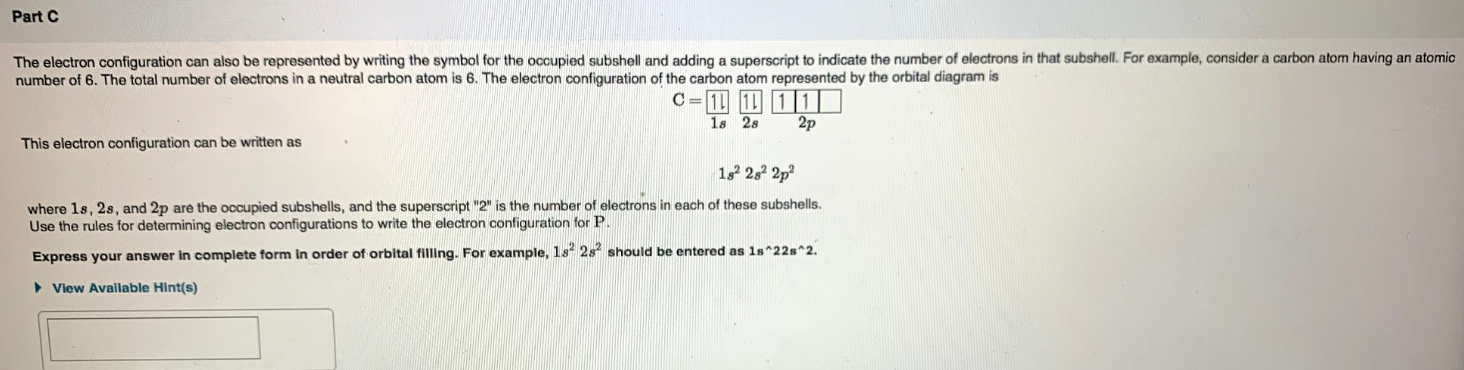# The electron configuration can also be represented by writing the symbol for the occupied subshell and adding a superscript to indicate the number of electrons in that subshell. For example, consider a carbon atom having an atomic number of 6. The total number of electrons in a neutral carbon atom is 6. The electron configuration of the carbon represented by the orbital diagram is. This electron configuration can be written as 1s^22s^22p^2 where 1s, 2s, and 2p are the occupied subshells, and the superscript "2" is the number of electrons in each of these subshells. Use the rules for determining electron configurations to write the electron configuration for P. Express your answer in complete form in order of orbital filling. For example, 1s^22s^2 should be entered as 1s^22s^2.